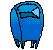Elementary Algebra
Assignment #1

Translate into a mathematical expression:

1. x increased by 7

2. the difference between x and y

3. the quotient of y and 3

4. the quotient when 4 is divided by x

5. the sum of x and y divided by the difference of x and y

6. ten less than x

7. the product of a, b, and c

8. the sum of 7 and x

9. x minus 8

10. x less than 8

11. The difference of 5 and x

12. the product of x and 9

13. 5 more than x

14. x decreased by 2

15. y less than 4### email